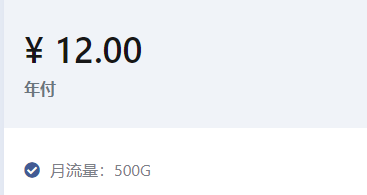• 累计撰写 171 篇文章
• 累计创建 3 个标签
• 累计收到 109 条评论

### 目 录CONTENT# 10-函数的类型.md2022-03-21 / 0 评论 / 0 点赞 / 467 阅读 / 3,516 字 / 正在检测是否收录...广告

# 函数的类型

## 函数声明§

// 函数声明（Function Declaration）
function sum(x, y) {
return x + y;
}

// 函数表达式（Function Expression）
let mySum = function (x, y) {
return x + y;
};


function sum(x: number, y: number): number {
return x + y;
}


function sum(x: number, y: number): number {
return x + y;
}
sum(1, 2, 3);

// index.ts(4,1): error TS2346: Supplied parameters do not match any signature of call target.
function sum(x: number, y: number): number {
return x + y;
}
sum(1);

// index.ts(4,1): error TS2346: Supplied parameters do not match any signature of call target.


## 函数表达式§

let mySum = function (x: number, y: number): number {
return x + y;
};


let mySum: (x: number, y: number) => number = function (x: number, y: number): number {
return x + y;
};


## 用接口定义函数的形状§

interface SearchFunc {
(source: string, subString: string): boolean;
}

let mySearch: SearchFunc;
mySearch = function(source: string, subString: string) {
return source.search(subString) !== -1;
}


## 可选参数§

function buildName(firstName: string, lastName?: string) {
if (lastName) {
return firstName + ' ' + lastName;
} else {
return firstName;
}
}
let tomcat = buildName('Tom', 'Cat');
let tom = buildName('Tom');


function buildName(firstName?: string, lastName: string) {
if (firstName) {
return firstName + ' ' + lastName;
} else {
return lastName;
}
}
let tomcat = buildName('Tom', 'Cat');
let tom = buildName(undefined, 'Tom');

// index.ts(1,40): error TS1016: A required parameter cannot follow an optional parameter.


## 参数默认值§

function buildName(firstName: string, lastName: string = 'Cat') {
return firstName + ' ' + lastName;
}
let tomcat = buildName('Tom', 'Cat');
let tom = buildName('Tom');


function buildName(firstName: string = 'Tom', lastName: string) {
return firstName + ' ' + lastName;
}
let tomcat = buildName('Tom', 'Cat');
let cat = buildName(undefined, 'Cat');


## 剩余参数§

ES6 中，可以使用 ...rest 的方式获取函数中的剩余参数（rest 参数）：

function push(array, ...items) {
items.forEach(function(item) {
array.push(item);
});
}

let a: any[] = [];
push(a, 1, 2, 3);


function push(array: any[], ...items: any[]) {
items.forEach(function(item) {
array.push(item);
});
}

let a = [];
push(a, 1, 2, 3);


## 重载§

function reverse(x: number | string): number | string {
if (typeof x === 'number') {
return Number(x.toString().split('').reverse().join(''));
} else if (typeof x === 'string') {
return x.split('').reverse().join('');
}
}


function reverse(x: number): number;
function reverse(x: string): string;
function reverse(x: number | string): number | string {
if (typeof x === 'number') {
return Number(x.toString().split('').reverse().join(''));
} else if (typeof x === 'string') {
return x.split('').reverse().join('');
}
}


## 参考§

0
1.2.广告
• 0
1.2.